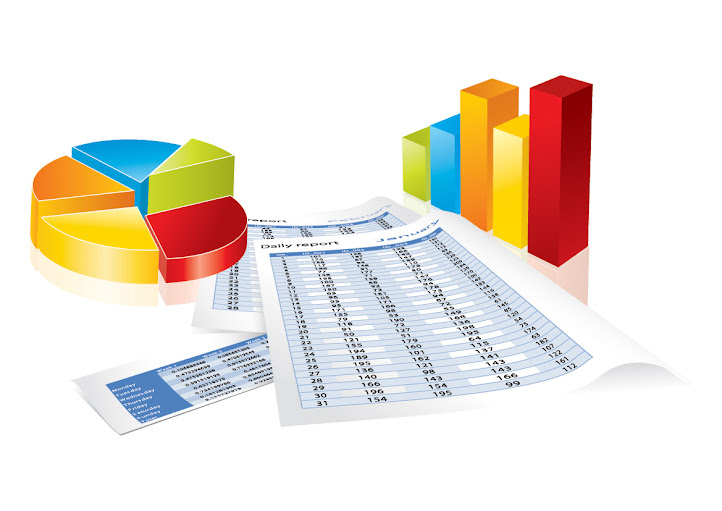Statistics

Statistics allow for informative decision-making formulated from theoretical and experimental data analysis. Data analysis has become so integrated into today’s modern lifestyles that most individuals are unaware of its role in their daily lives. This can be seen in reality shows that base results upon contestants’ national popularity and voting results. Box office movie rankings are based on weekend ticket sales. At an individual level, people use statistics for calculating gas mileage, green calculations such as average energy consumption, insurance rates, and calculating class rank and GPA.

In this lesson students will learn the processes of gathering, organizing, interpreting, and formulating an understanding of data through probability and statistics.

K1 – Name measures of central tendency and variation and describe their meaning.

S1 – Calculate the theoretical probability that a simple event will occur.
S2 – Produce a frequency distribution to describe experimental results and create a histogram to communicate these results.
S3 – Calculate the probability of making a set of observations in a series of trials where each trial has two distinct possible outcomes.
S4 – Apply AND, OR, and NOT logic to probability.
S5 – Apply Bayes’ Theorem to calculate a probability in a manufacturing context.
S6 – Calculate the central tendency of a data set, including mean, median, and mode.
S7 – Calculate the variation in a set of data, including range, standard deviation, and variance.

Resources

Vandegrift High School | 9500 McNeil Drive, Austin, TX | T: 512 570 2300 |  Andrew.Perrone@leanderisd.org